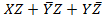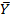# A voltage signal 10 sin ωt is applied to the circuit with ideal diodes as shown in figure,the maximum and minimum values of the output waveform Vout of the circuit are respectively 10 kwΩ

1.  +10 V and -10 V

2.  +4 V and -4 V

3.  +7 V and -4V

4.  +4 V and -7 V

4

+4 V and -4 V

Explanation :
No Explanation available for this question

# The circuit of figure shows a 555 timer IC connected as an multivibrator.The value of the capacitor C is 10 nF.The values of the resistors RA and RB for a frequency of 10 kHz and duty cycle of 0.75 for the output voltage waveform are

1.  RA=3.62 kΩ,RB=3.62 kΩ

2.   RA=3.62 kΩ,RB=7.25 kΩ

3.  RA=7.25 kΩ,RB=3.62 kΩ

4.   RA=7.25 kΩ,RB=7.25 kΩ

4

RA=7.25 kΩ,RB=3.62 kΩ

Explanation :
No Explanation available for this question

# The simplified block diagram of a 10-bit A/D converter of dual slope integrator type is shown in the figure.The 10-bit counter at the output is clocked by a 1 MHz clock.Assuming negligible timing overhead for the control logic,the maximum frequency of the analog signal that can be converted using this A/D converter is approximately

1.  2 kHz

2.  1  kHz

3.  500 Hz

4.  250 Hz

4

1  kHz

Explanation :
No Explanation available for this question

# The Boolean expression can be simplified to

1.

2.

3.

4.

4Explanation :
No Explanation available for this question

# The shift register shown in the figure is initially loaded with the bit pattern 1010.subsequently the shift register is clocked and with each clock pulse the pattern gets shifted by one bit position to the rught.With each shift,the bit at the serial input is pushed to the left most position (msb),After how many clock pulses will the content of the shift register become 1010 again

1.  3

2.  7

3.  11

4.  15

4

7

Explanation :
No Explanation available for this question

# An X-Yflip flop,whose characteristic table is given below is to be implemented using a J-K flip flop X Y Qn+1 0 0 1 0 1 Qn 1 0 Qn 1 1 0 This can be done by making

1.  J=X,K=

2.  J= ,K=Y

3.  J=Y,K=

4.  J= ,K=X

4

J=,K=X

Explanation :
No Explanation available for this question

# A memory system has a total of 8 memory chips,each with 12 address lines and 4 data lines.The total size of the memory system is

1.  6 kbytes

2.  32 kbytes

3.  48 kbytes

4.  64 kbytes

4

32 kbytes

Explanation :
No Explanation available for this question

# The following program is written for an 8085 microprocessor to add two bytes located at memory addresses 1FFE and 1FFF LXI H.1FFE MOV B,M INR L MOV A,M ADD B INR L MOV M,A XOR A On completion of the execution of the program the result of addition is found

1.  in the register A

2.  at the memory address 1000

3.  at the memory address 1F100

4.  at the memory address 2000

4

Explanation :
No Explanation available for this question

# A control system with certain excitation is governed by the following mathematical equation .The natural time constants of the response of the system are

1.  2s and 5s

2.  3s and 6s

3.  4s and 5s

4.  1/3s and 1/6s

4

3s and 6s

Explanation :
No Explanation available for this question

1.  25%

2.  0.75%

3.  6%

4.  33%

4